# Electronics and Communication Engineering - Exam Questions Papers

1.
It is desired to design a phase shift oscillator using an FET having a gm = 5000 μSiemen, rd = 40 kΩ and feedback circuit value of R = 10 K. Then value of C for oscillator operation at 1 kHz and that of RD for A = 40 to ensure oscillator action are
6.4 nF, 80 K
6.5 nF, 10 K
6.5 pF, 10 K
65 pF, 80 K
Explanation:

For a FET oscillator, the oscillator frequency is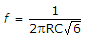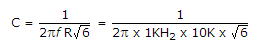C = 6.4 nF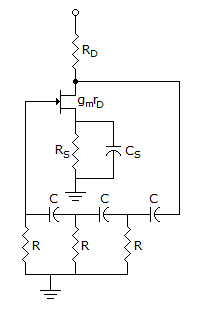For FET Oscillator,

|A| = gmRL = 5000 x 10-6 RL

i.e., 40 = 5000 x 10-6 x RL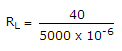= 8 KΩ

Now,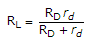Using RL = 8 K and rd = 40 K (given)

We find RD as follows: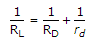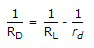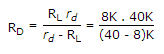= 10 K

RD = 10 K.

2.
The type of feedback in the following circuit diagram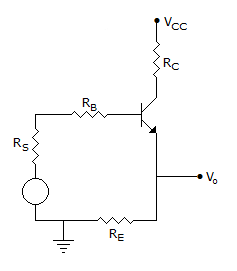Voltage shunt
Current-shunt
Voltage series
Current series
Explanation:

Output is taken directly from feedback and feedback path is in series with input. Thus it is voltage series.

3.
Modem warfare has changed from large scale clashes of armies to suppression of civilian populations. Chemical agents that do their work silently appear to be suited to such warfare; and regretfully, there exist, people in military establishments who think that chemical agents are useful tools for their cause, which of the following statements best sums up the meaning of the above passage:
Modem warfare has resulted in civil strife.
Chemical agents are useful in modem warfare.
Use of chemical agents in warfare would be undesirable.
People in military establishments like to use chemical agents in war.
Explanation:

Among the answer choices, the three options B, C and D can be inferred from the passage. But the main essence of the passage is that chemical agents are being used my military establishments in warfare which is not desirable. Therefore option C is the statement which best sums up the meaning of the passage.

4.
The equation for loop currents I1 and I2 are __________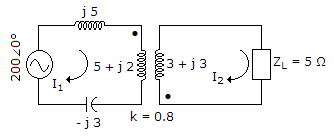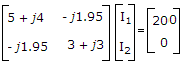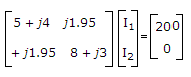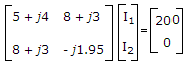Explanation:

k = 0.8

M = kL1L2 = 0.83 x 2 = 1.95 H

Apply KVL to loop 1

200∠0° = (j5 + 5 + j2 - j3)I1 + 1.95I2

200∠0° = (5 + j4)I1 + j1.95I2

Apply KVL to loop 2

0 = (5 + 3 + j3)I2 + j1.95I1

0 = (8 + j3)I2 + j1.95I1

0 = j1.95I1 + (8 + j3)I2

The loop equations in matrix form are5.
A power of 100 W is radiated from an isotropic radiator. The power radiated peer unit solid angle and the power density at a distance of 10 km from the antenna is respectively
25 W, 10 W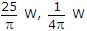7.96 W, 0.08 μW
0.08 μ W, 7.96 W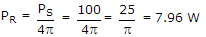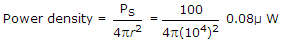.